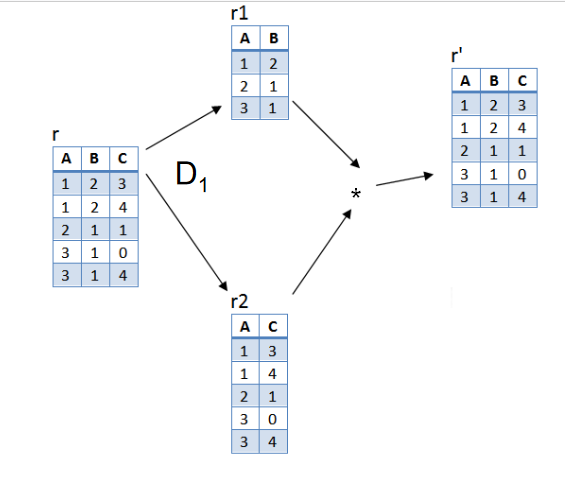# Relational decomposition and dependency preserving

### Relational decomposition

1. When a relation in the relational model is not in appropriate normal form then the decomposition of a relation is required.
2. In a database, it breaks the table into multiple tables
3. If the relation has no proper decomposition, then it may lead to problem like loss of information.
4. Decomposition is used to eliminate some of the problems of bad design like anomalies, inconsistency, and redundancy ext.

Two types of  decomposition's are there

1. Lossless  decomposition / nonadditive  decomposition

2. Lossy decomposition / additive decomposition

We will discuss one by one with examples in detail

1. Lossless  decomposition / nonadditive  decomposition

1.  If the information is not lost from the relation that is decomposed, then the decomposition will be lossless.
2. The lossless decomposition guarantees that the join of relations will result in the same relation as it was not decomposed.
3. The relation is said to be lossless decomposition if natural join of all the decomposition gives the original relation.

Example:-

Consider the relation R(ABC) and it is decomposed into R1(AB) and R2(BC)

In the above example, the natural join result is not same as original relation R, so it is lossy.

2. Lossless join Decomposition / additive decomposition

Consider the relation R(ABC) and it is decomposed into R1(AB) and R2(AC)In the above example, the natural join result is same as original relation R, so it is loss less.

From the above two examples, we can conclude that

1. If  R1 ∩ R2 = Φ ( that is no common attribute) then it is lossy
2. If  R1 ∩ R2 ≠ Φ and common attribute is not a key of either R1 or R2 it is lossy
3. If R1 ∩ R2 ≠ Φ and R1 ∩ R2 results either as R1 or R2 and common attribute is a key of either R1 or R2 decomposed relations, then it is loss less.

### Dependency preserving

It is an important constraint of the database.

In  the dependency preservation, at least one decomposed table must satisfy every dependency.

If a relation 'R' is decomposed into relation R1 and R2, then the dependencies either must be a part of R1 or R2 or must be derivable from the combination of FD's of R1 and R2

Example:-

Suppose R (A B C D) and set of functional dependencies
F: AB->CD, D-> A
If R is decomposed into following two relations
R1 (A D),
R2 ( B C D)

Identify that this decomposition is dependency preserving or not?

Solution:

R (A B C D)

F: AB->CD, D-> A

It can be seen that while decomposing, the functional dependency AB->CD has been
lost.
To understand, calculate AB + = AB [ Using FD in F` ] while in F, AB can determine CD
but this dependency is lost in F`.
Therefore, this decomposition is not dependency preserving decomposition

More

More

MFCS
COA
PL-CG
DBMS
OPERATING SYSTEM
SOFTWARE ENG
DSA
TOC-CD
ARTIFICIAL INT

More

More

More

More
Top